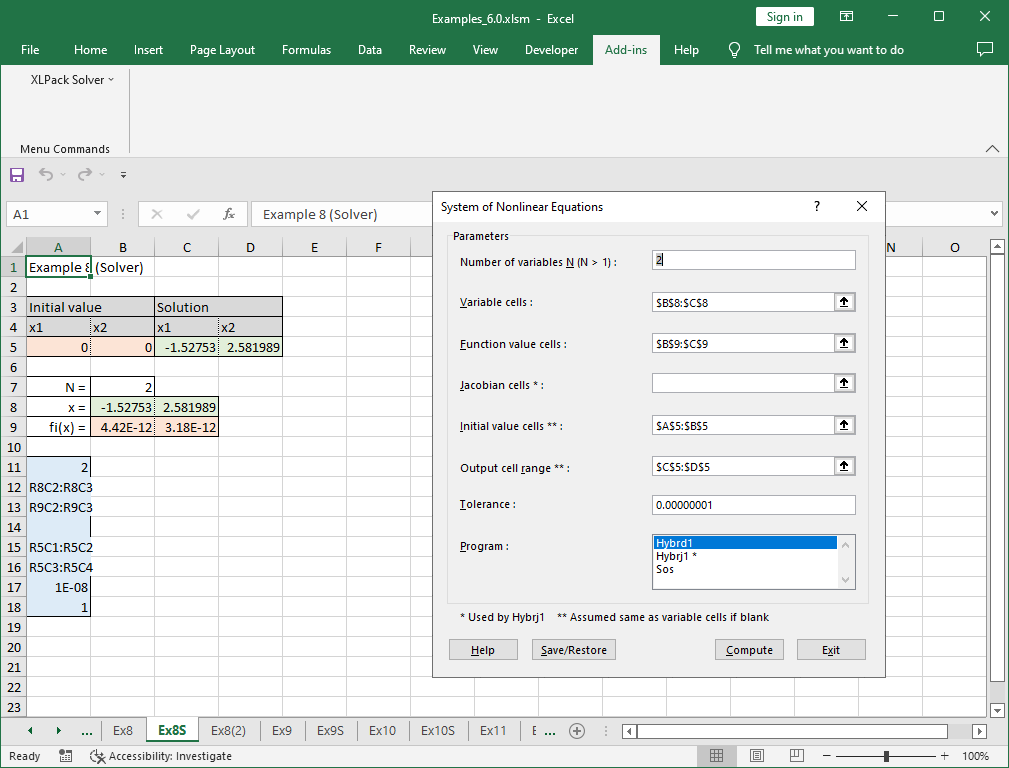# 1. How to obtain and use example worksheets

## Where to obtain example worksheets

For those who want to run the examples used in this tutorial, the example worksheets are available below.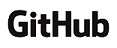(Open page in a new tab)

Note – For your reference, other sample worksheets using more functions are also available below (Not examples of this tutorial).

## Preparation for using example worksheets

The software requirements required to use the example worksheets are as follows.

• Windows 10 (Version 2004 or later) or Windows 11 is running
• Office 365, 2021, 2019, 2016 or 2013 (Excel) is installed

If these requirements are met, follow the instructions in User’s Guide to install and set up the required software.

• Install “XLPack Basic” (User’s Guide Section 3.)

## How to use example worksheets

### Structure of example book

The example book contains three types of example worksheets: A worksheet using worksheet functions, a worksheet using VBA programs, and a worksheet using solver.

Worksheet name consists of “Ex + number + symbol”. The number is the chapter number of tutorial. The symbol is “W” for a worksheet using worksheet functions, or “S” for a worksheet using solver. If there is no symbol, it is a worksheet using VBA programs.

Example:

Ex2W – The example worksheet for “2. System of linear equations” using worksheet functions
Ex2 – The example worksheet for “2. System of linear equations” using VBA programs
Ex8S – The example worksheet for “8. System of nonlinear equations” using solver

Module name containing VBA programs is also “Ex + number”. The number is the chapter number.

### How to use example worksheets using worksheet functions

Let’s explain using the example for “2. System of linear equations” shown below.

#### Example

We will solve the following linear equations.

```
10x1 - 7x2 = 7
-3x1 + 2x2 + 6x3 = 4
5x1 - x2 + 5x3 = 6

```

These equations are conveniently written in the matrix form.

```
Ax = b

```

where,

```
( 10 -7  0 )        ( 7 )        ( x1 )
A = ( -3  2  6 ),   b = ( 4 ),   x = ( x2 )
(  5 -1  5 )        ( 6 )        ( x3 )

```

A is the coefficient matrix, b is the right-hand side vector, and x is the solution vector.

In the example worksheets, orange cells represent the cells where the data (and/or sometimes formulas) are entered, and green cells represent the cells where the worksheet functions are to be entered.

Although the worksheet functions are already entered in the green cells of downloaded example worksheet, let’s explain from the beginning.

Enter the data of coefficient matrix A and right-hand side vector b into the appropriate cells (orange cells) in the worksheet, and select (order of the equations + 2) cells where the solution vector is to be placed (green cells).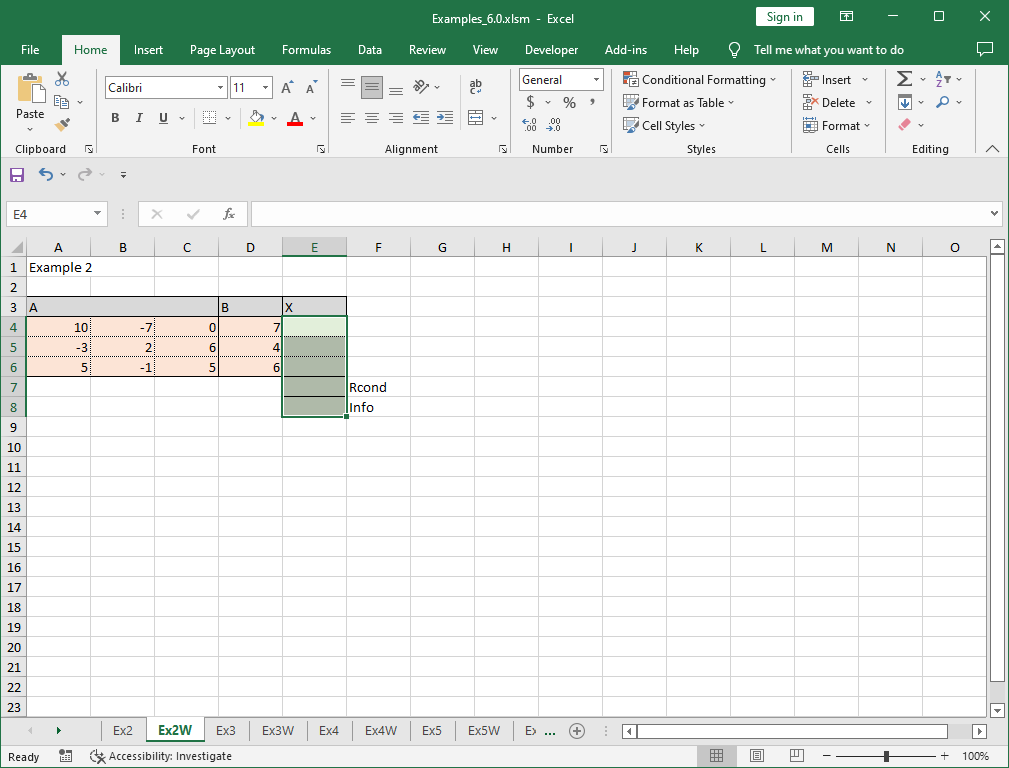Click fx in the formula bar. Then select WDgesv worksheet function from category XLPack.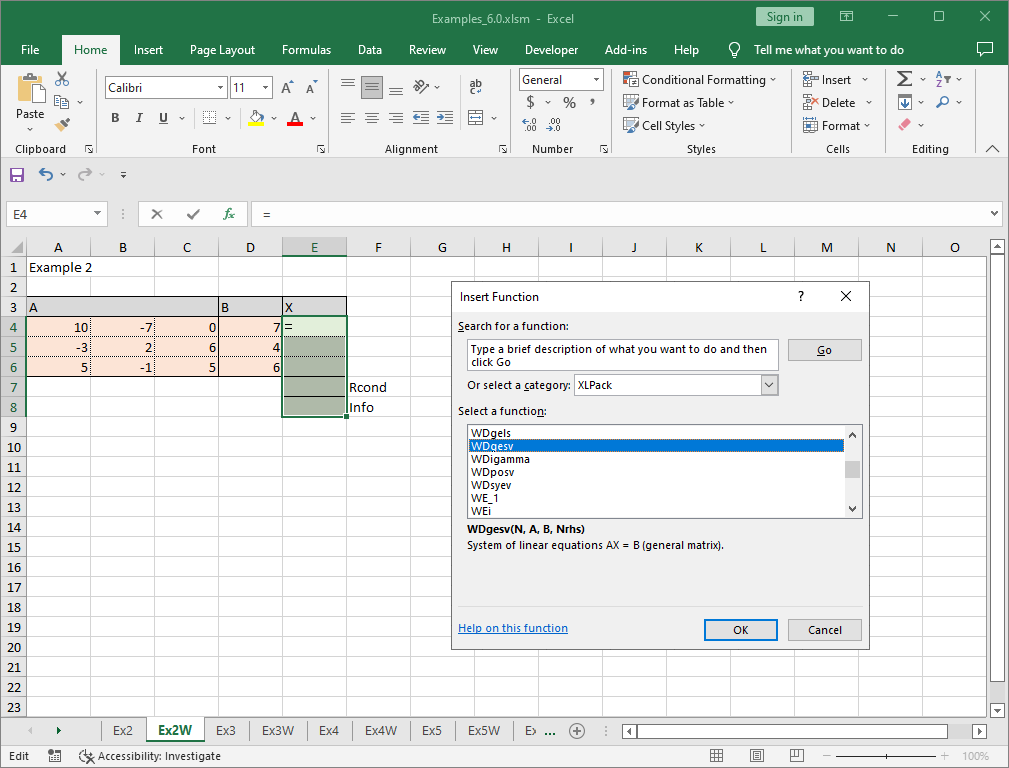Supply the appropriate values for the requested parameters N (order of the equations, e.g. 3), A (range of coefficient matrix) and B (range of right-hand side vector). More than one right-hand side vectors can be entered and Nrhs is the number of right-hand side vectors. Nrhs can be omitted, in which case it is assumed to be one.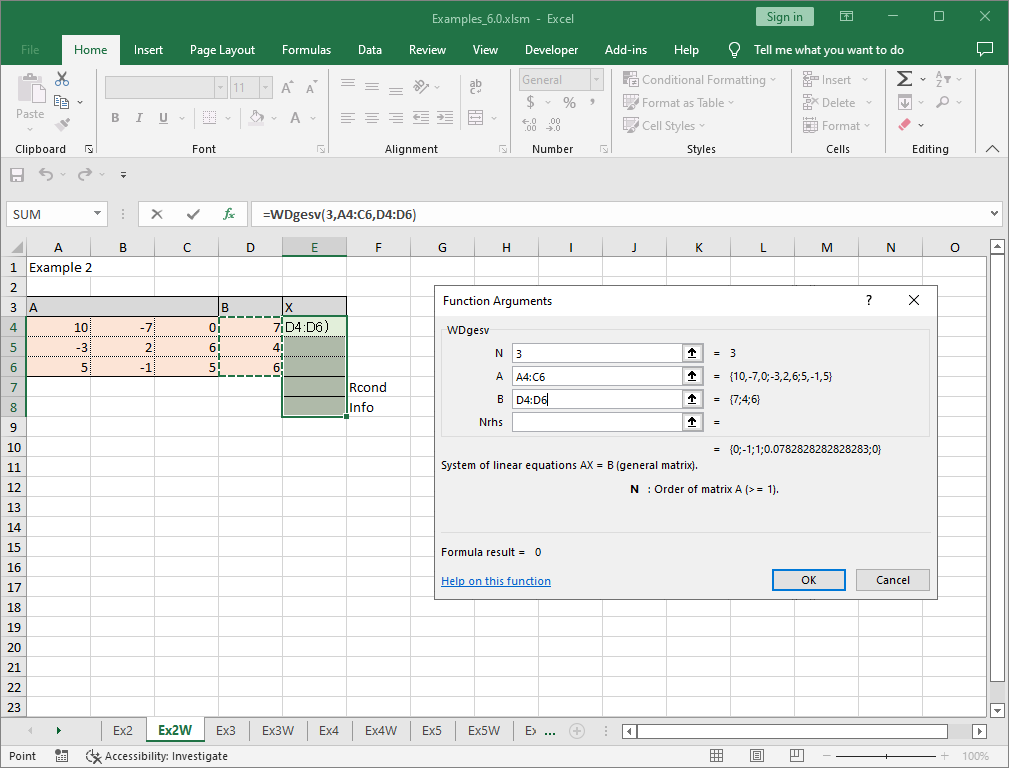Then press Ctrl + Shift + Enter.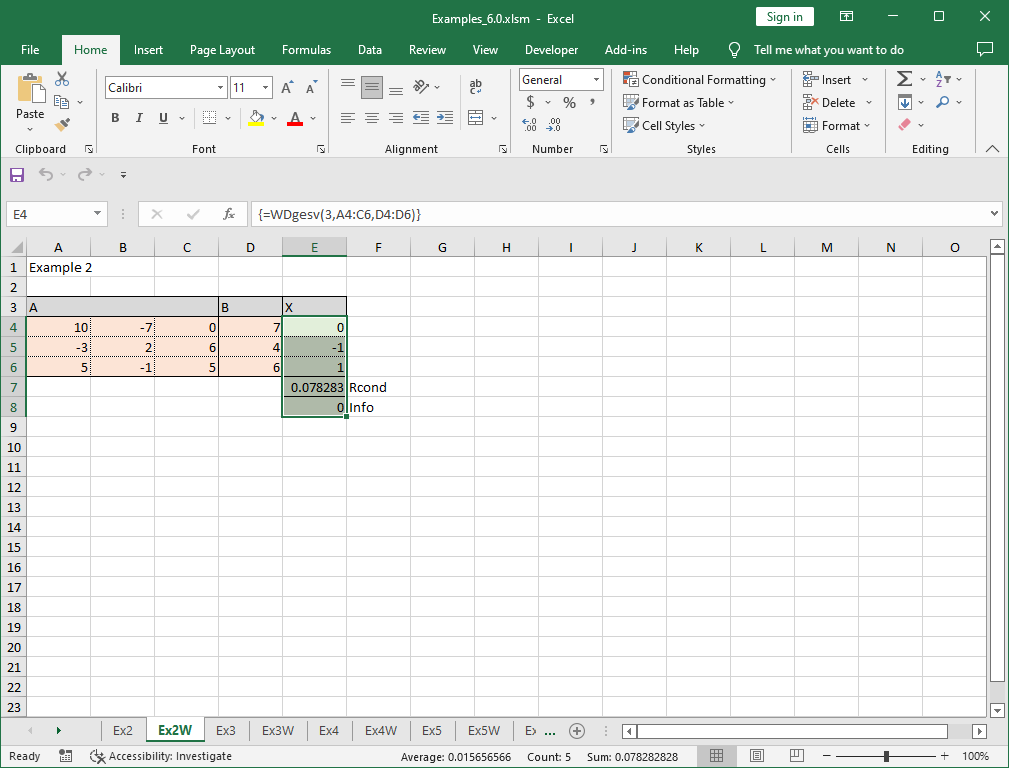Now, we’ve got the solution X=(0 -1 1)T. The next cell with the value of 0.078283 is the reciprocal of the the estimated condition number and the undermost cell with the value 0 is the return code (0 shows normal return). We selected extra 2 cells for displaying these information. You may select only the cells for the solution (3 cells in this example) if such information is not necessary.

### How to use example worksheets using VBA programs

Let’s explain using the example for “2. System of linear equations” as above.

In the example worksheets, orange cells represent the cells where the data (and/or sometimes formulas) are entered, and green cells represent the cells to be wrtten by the VBA programs.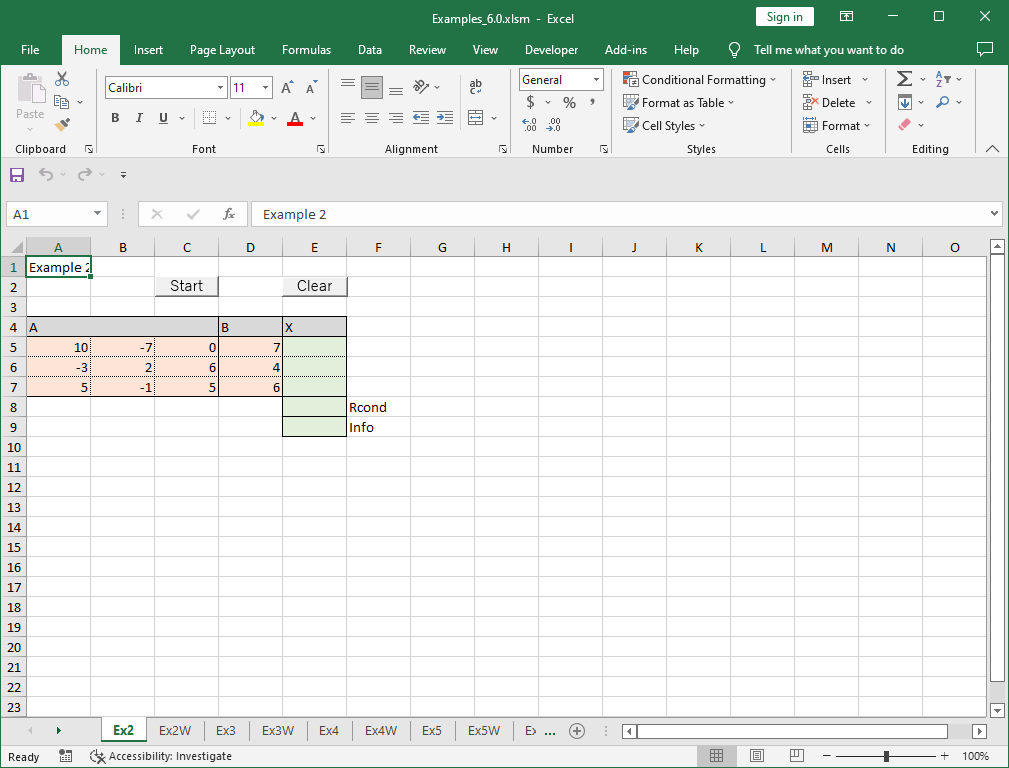The program can be found in module Ex2.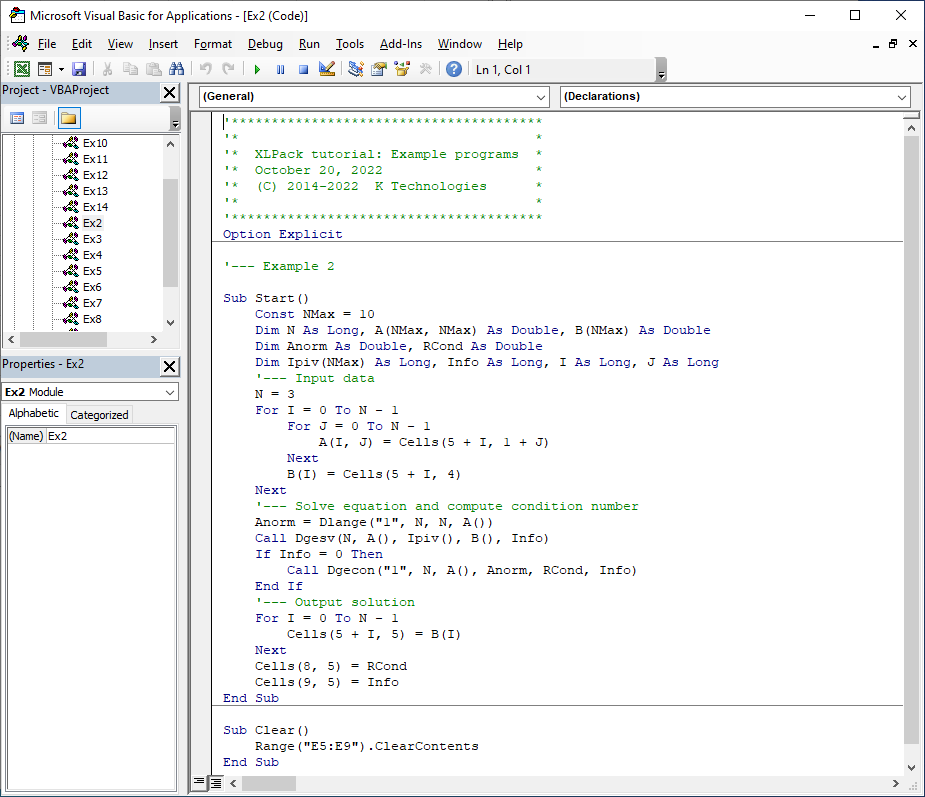To run program, click “Developer” -> “Macros”, choose Ex2.Start, and click “Run”. In this worksheet, Start macro is assigned to “Start” button so that you can also run program simply by clicking it.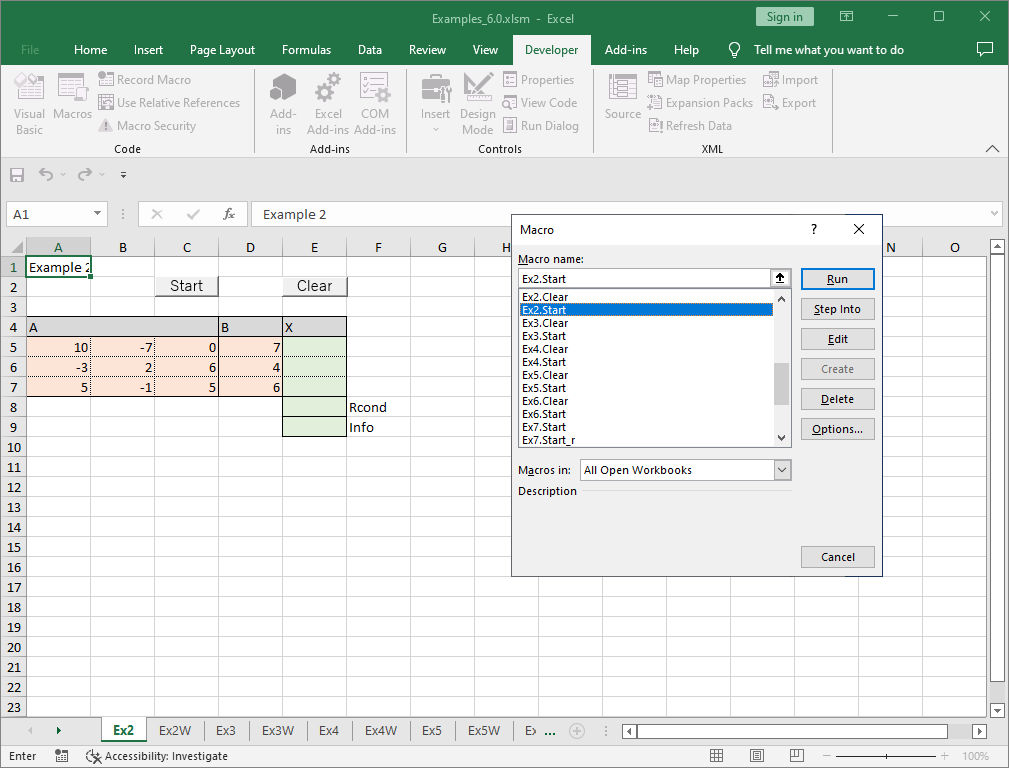The results are written in green cells.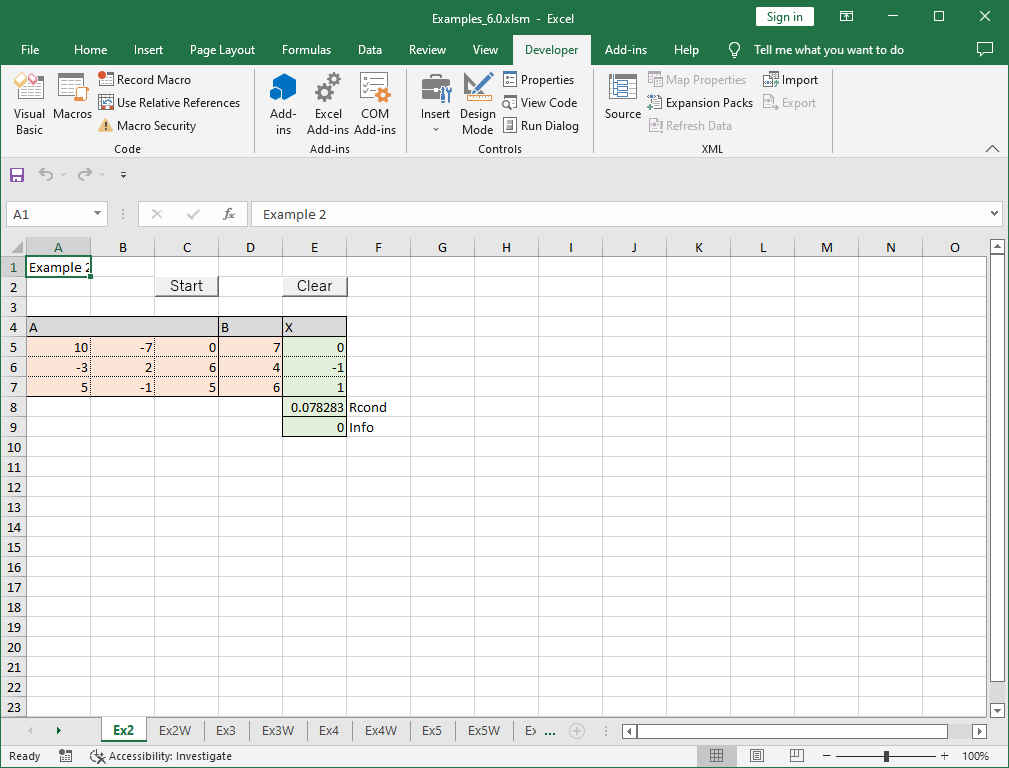The macro which clear the green cells is assigned to “Clear” button.

### How to use example worksheets using solver

Let’s explain using the example for “8. System of nonlinear equations” shown below.

#### Example

We will solve the following nonlinear equations.

```
f1(x1, x2) = 4x12 + x22 - 16 = 0
f2(x1, x2) = x12 + x22 - 9 = 0

```

In the example worksheets, orange cells represent the cells where the data (and/or sometimes formulas) are entered, green cells represent the cells to be wrtten by the solver, and blue cells represent the parameter save area for solver.

Cells A5 and B5 are used as variales x1 and x2, respectively. The first equation (=4*B8^2+C8^2-16) is storeed in the cell B9, and the second equation (=B8^2+C8^2-9) is storeded in the cell C9.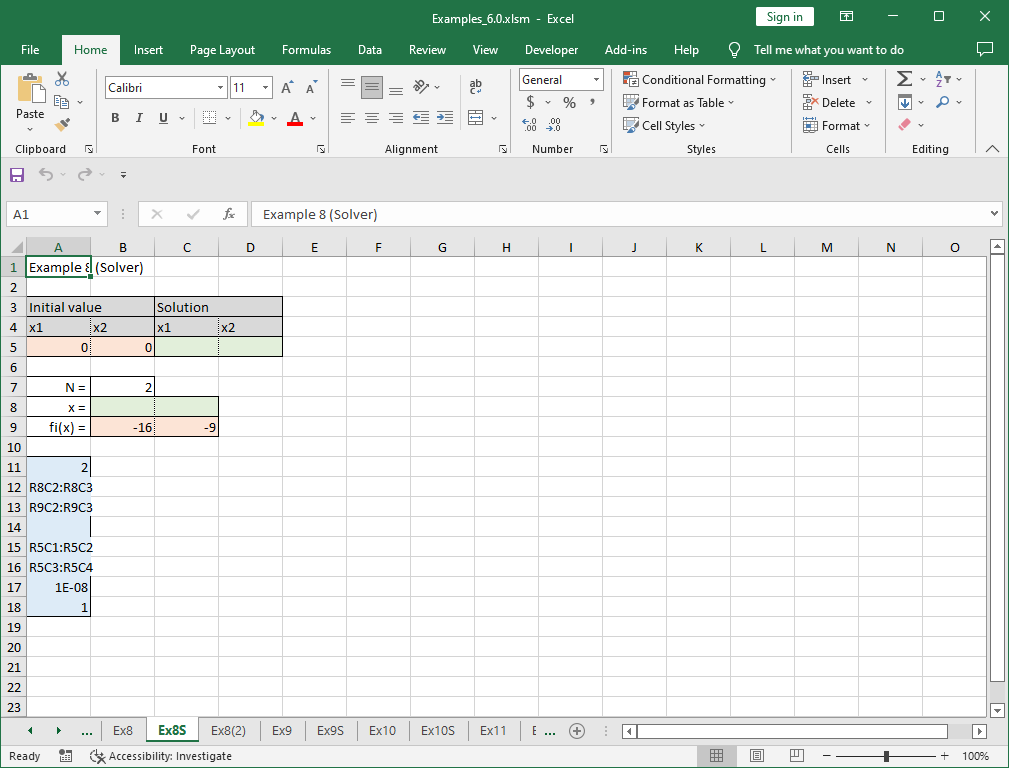Click “Add-ins” -> “XLPack Solver” -> “System of Nonlinear Equations”.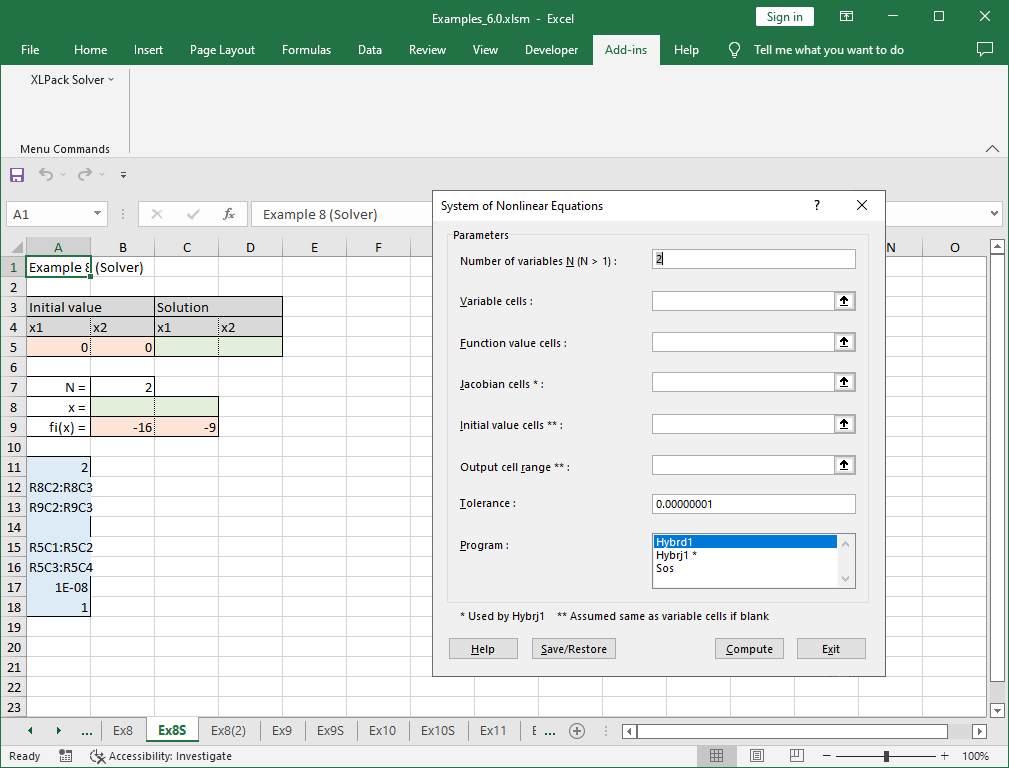Click “Save/Restore”.

Choose blue parameter save area and click “Restore”.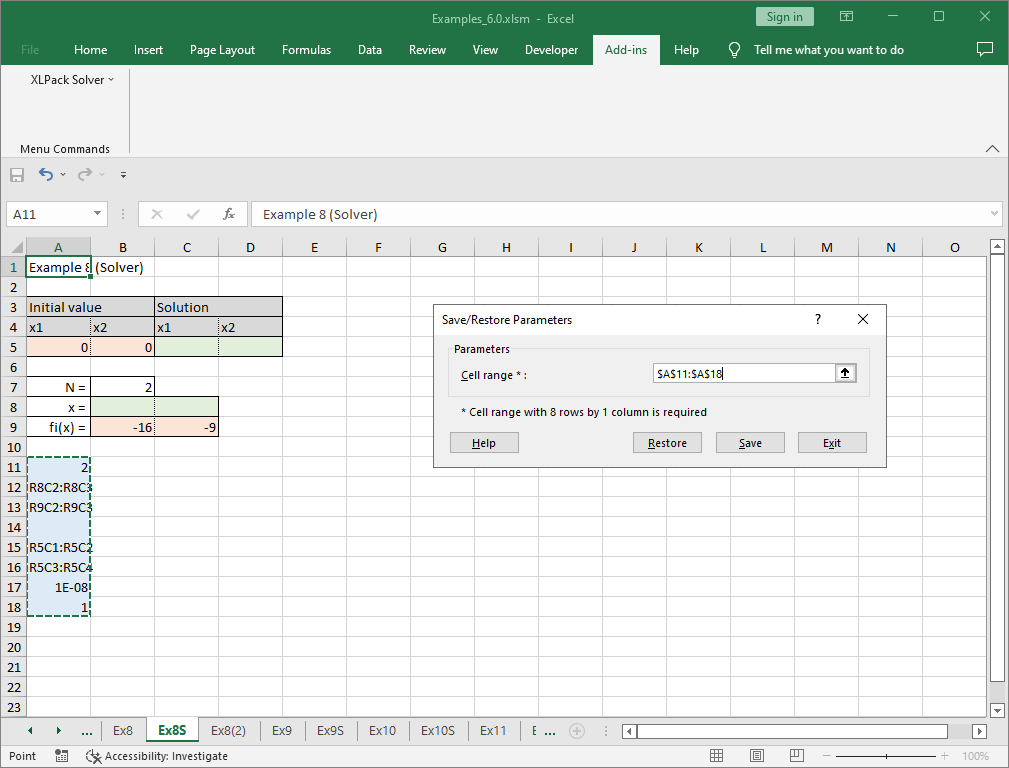The required parameters are set in the solver.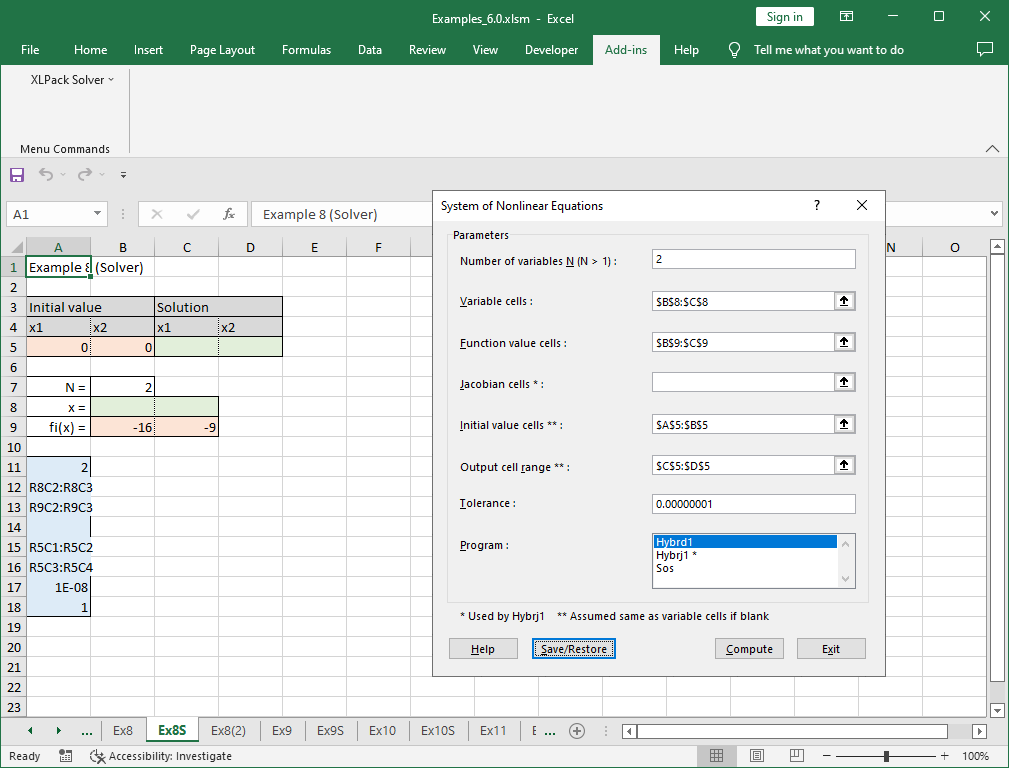By clicking “Compute” the calculation starts and the result will be written in the green cells.